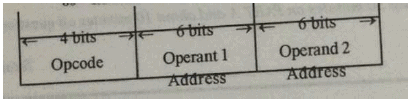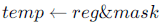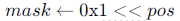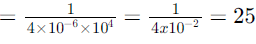# Test: Instructions & Input Output Interface

## 15 Questions MCQ Test RRB JE for Computer Science Engineering | Test: Instructions & Input Output Interface

Description
Attempt Test: Instructions & Input Output Interface | 15 questions in 45 minutes | Mock test for Computer Science Engineering (CSE) preparation | Free important questions MCQ to study RRB JE for Computer Science Engineering for Computer Science Engineering (CSE) Exam | Download free PDF with solutions
QUESTION: 1

### Using an expanding opcode encoding for instructions, is it possible to encode all of the following in an instruction format shown in the below figure. Justify your answer. 14 double address instructions 127 single address instructions 60 no address (zero address) instructionsSolution:

4 bits are for the opcode so number of 2 address instructions will be 24 =16 ---so 14 double instructions are possible
But out of 16 only 14 are used so 2 are still left which can be used for 1 address instruction
For 1 address instruction we can use not only the 2 left over but also the 6 bits of operand1(to make it one address)--- so
6 bits that is 64 so they are laready 2 which each contains 64 so total 128 single address instructions -----so 127 single
instructions are possible
But out of 128 ,127 are used so 1 left which can be used for zero address instruction to make number of zero address we
can use the operand2 address 6 bits so total possible are 64 so total 1*64 = 64 zero address instructions are possible
So all encoding are possible

QUESTION: 2

### Which of the following statements is true?

Solution:

It is C.
Only the top of the stack can be accessed at any time. You can imagine a stack to be opened from only one side data
structure. So that if we put one thing over the other, we are able to access the last thing we inserted first. That is Last in

First Out (LIFO).
PC points to the next instruction to be executed.
Not all instructions affect the flags.

QUESTION: 3

### Which of the following is not a form of memory

Solution:

The instruction opcode is a part of the instruction which tells the processor what operation is to be performed so it is not a form of memory while the others are

QUESTION: 4

Consider a new instruction named branch-on-bit-set (mnemonic bbs). The instruction “bbs reg, pos, label” jumps to label if bit in position pos of register operand reg is one. A register is 32 bits wide and the bits are numbered 0 to 31, bit in position 0 being the least significant. Consider the following emulation of this instruction on a processor that does not have bbs implemented.Branch to label if temp is non-zero. The variable temp is a temporary register. For correct emulation, the variable mask must be generated by

Solution:We want to check for a particular bit position say 2 (third from right). Let the number be 0xA2A7 (last 4 bits being 0111).
Here, the bit at position 2 from right is 1. So, we have to AND this with 0x0004 as any other flag would give wrong value
(may count other bits or discard the bit at position "pos"). And 0x0004 is obtained by 0x1 << 2 (by shifting 1 "pos" times
to the left we get a flag with 1 being set only for the "pos" bit position).

QUESTION: 5

Consider a RISC machine where each instruction is exactly 4 bytes long. Conditional and unconditional branch instructions use PC-relative addressing mode with Offset specified in bytes to the target location of the branch instruction. Further the Offset is always with respect to the address of the next instruction in the program sequence. Consider the following
instruction sequence Instr. No. Instruction
i : add R2, R3, R4
i + 1 : sub R5, R6, R7
i + 2 : cmp R1, R9, R10
i + 3 : beq R1, Offset
If the target of the branch instruction is i, then the decimal value of the Offset is ____________

Solution:

Ans:(-16)
2004--sub r5,r6,r7
2008---r1,r9,r10
2012--beq r1,offset //pc after instruction fetch of this instruction will be 2016,and branch target is 2000 ,offset will be (2016-16) = 2000
2016------next instruction

QUESTION: 6

Consider a processor with 64 registers and an instruction set of size twelve. Each instruction has five distinct fields, namely, opcode, two source register identifiers, one destination register identifier, and twelve-bit immediate value. Each instruction must be stored in memory in a byte-aligned fashion. If a program has 100 instructions, the amount of memory (in bytes)
consumed by the program text is _________.

Solution:

Number of register = 64
Number of bits to address register = [log264] = 6 bits
Number of Instruction = 12
Opcode size = [log212] = 4
Opcode(4) reg1(6) reg2(6) reg2(6) Immediate(12)
Total bits per instruction = 34
Total bytes per instruction= 4.25
Due to byte alignment we cannot store 4.25 bytes, without wasting 0.75 bytes ,
So Total bytes per instruction = 5
Total instruction = 100
Total size = Number of instruction x Size of instruction 100 x 5= 500 Byes

QUESTION: 7

In an 11-bit computer instruction format, the size of address field is 4-bits. The computer uses expanding OP code technique and has 5 two-address instructions and 32 one-address instructions. The number of zero-address instructions it can support is ________

Solution:

No. of possible instruction encoding = 211 = 2048
No. of encoding taken by two-address instructions = 5 × 24 × 24 = 1280
No. of encoding taken by one-address instructions = 32 × 24 = 512
So, no. of possible zero-address instructions = 2048 − (1280 + 512) = 256

QUESTION: 8

State True or False with one line explanation Expanding opcode instruction formats are commonly employed in RISC. (Reduced Instruction Set Computers) machines.

Solution:

RISC systems use fixed length instruction to simplify pipeline.
eg: MIPS, PowerPC: Instructions are 4 bytes long.
CISC systems use Variable-length instructions.
eg: Intel 80x86: Instructions vary from 1 to 17 bytes long.
Now the challenge is: How to fit multiple sets of instruction types into same (limited) number of bits (Fixed size instruction)?
Here comes Expanding opcode into the picture.
RISC systems commonly uses Expanding opcode technique to have fixed size instructions.

QUESTION: 9

A machine has a 32-bit architecture, with 1-word long instructions. It has 64 registers, each of which is 32 bits long. It needs to support 45 instructions, which have an immediate operand in addition to two register operands. Assuming that the immediate operand is an unsigned integer, the maximum value of the immediate operand is ____________

Solution:
QUESTION: 10

Many of the advanced microprocessors prefetch instructions and store it in an instruction buffer to speed up processing. This speed up is achieved because ________

Solution:
QUESTION: 11

On receiving an interrupt from a I/O device the CPU:

Solution:

Answer should be D i.e branches off to ISR after completing current instruction.
CPU checks the status bit of interrupt at the completion of each current instruction running when there is a interrupt it service the interrupt using ISR.

QUESTION: 12

In a vectored interrupt

Solution:

A vectored interrupt is a processing technique in which the interrupting device directs the processor to the appropriate interrupt service routine. This is in contrast to a polled interrupt system, in which a single interrupt service routine must determine the source of the interrupt by checking all potential interrupt sources, a slow and relatively laborious process.

QUESTION: 13

Which of the following is true?

Solution:

Ans is A.
Options B and D is obviously false.
A processor checks for the interrupt before FETCHING an instruction, So Option C is also false.

QUESTION: 14

A device employing INTR line for device interrupt puts the CALL instruction on the data bus while

Solution:

INTR is a signal which if enabled then microprocessor has interrupt enabled it receives high INR signal & activates INTA signal, so another request can’t be accepted till CPU is busy in servicing interrupt. Hence (A) is correct option.

QUESTION: 15

A device with data transfer rate 10 KB/sec is connected to a CPU. Data is transferred byte-wise. Let the interrupt overheadbe 4 sec. The byte transfer time between the device interface register and CPU or memory is negligible. What is theminimum performance gain of operating the device under interrupt mode over operating it under program-controlled mode?

Solution:

In Programmed I/O, the CPU issues a command and waits for I/O operations to complete.
So here, CPU will wait for 1sec to transfer 10KB of data.
overhead in programmed I/O = 1 sec
In Interrupt mode , data is transferred word by word (here word size is 1 byte as mentioned in question "Data is transferred byte-wise").
So to transfer 1 byte of data overhead is 4 x 10-6sec
Thus to transfer 10 KB of data overhead is = 4 x 10-6 x 10sec
Performance gainUse Code STAYHOME200 and get INR 200 additional OFF Use Coupon Code